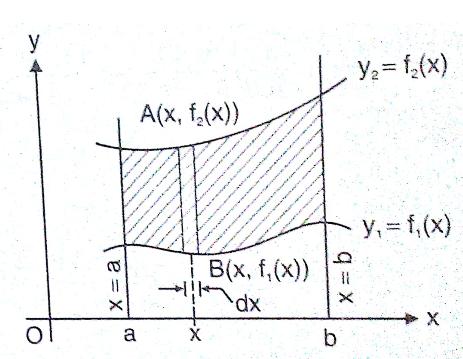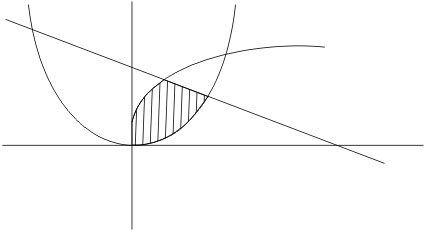# The area (in sq. units) of the regionis : Option 1)Option 2)Option 3)Option 4)As learnt in

Area along x axis -

Letbe two curve then area bounded between the curves and the lines

x = a and x = b is- whereinWherePoint of intersection ofOption 1)Incorrect option

Option 2)Incorrect option

Option 3)Correct option

Option 4)Incorrect option